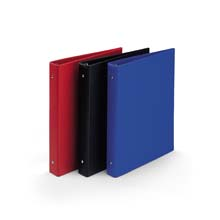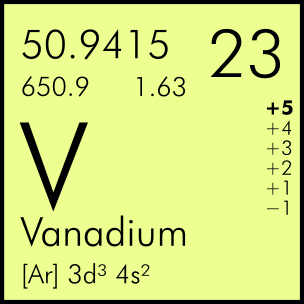` Wiskundeleraar

## Drieëntwintig23

• 23 is een priemgetal
• 11111111111111111111111 is ook een priemgetal
• 23 is het kleinste priemgetal waarvan zowel het voorgaande als het volgende oneven getal geen priemgetal is
..of dit bijzonder is weet ik nog niet:

232+233=23·3·232

Maar daarover later meer...

23 is the smallest number of integer-sided boxes that tile a box so that no two boxes share a common length...

23

XXIII

viginti tria

vicesimus tertius

• 23 has the unique representation 23 = 12+22+32+32 as a sum of 4 squares.
• 23 is a Germain prime, since 2×23+1 = 47 is also prime.
• control-W
ASCII: 23 (hex: 17 | octal: 0027 | binary: 00010111)
Unicode: U+0017 (decimal: 23)
(basic Latin)

Wolfram Alpha\$23^{23}\$=\$20880467999847912034355032910567\$ en dat bestaat uit 23 cijfers...((2*3)*4)-1 = 23
((2*4)*3)-1 = 23
((3*2)*4)-1 = 23
((3*4)*2)-1 = 23
((4*2)*3)-1 = 23
((4*3)*2)-1 = 23

Zie ook

In het artikel 'Weg van Getallen' staat:

• "Het aantal partities van alle 115000-vouden plus 3474 deelbaar zijn door 23.".

The sum of the first 23 primes is 874, which is divisible by 23, a property shared by few other numbers.